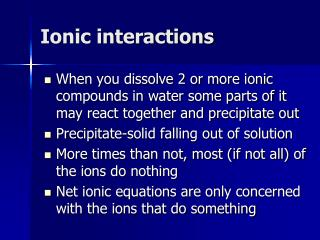# Ionic interactions - PowerPoint PPT PresentationDownload PresentationIonic interactions

Ionic interactions
Download Presentation## Ionic interactions

- - - - - - - - - - - - - - - - - - - - - - - - - - - E N D - - - - - - - - - - - - - - - - - - - - - - - - - - -
##### Presentation Transcript

1. Ionic interactions • When you dissolve 2 or more ionic compounds in water some parts of it may react together and precipitate out • Precipitate-solid falling out of solution • More times than not, most (if not all) of the ions do nothing • Net ionic equations are only concerned with the ions that do something

2. This goes back to ionic dissociation in water NaCl(s) + AgNO3(s)in water Na+(aq)+ Cl-(aq) + Ag+(aq) + NO3-(aq) Net ionic ignores everything that doesn’t do anything! AgCl(s) + Na+(aq)+ NO3-(aq) Ag+(aq) +Cl-(aq) AgCl(s) net ionic equation-- Here’s the trick, AgCl is insoluble

3. More net ionic equ. • You normally write the reactants as aqueous (since you are dissolving them) • Mg(NO3)2(aq) + Na2CO3(aq) MgCO3(s) + 2NaNO3(aq) • Dissociate everything that is aqueous, not solid • Mg++ + 2NO3- + 2Na+ + CO3-- •  MgCO3(s) + 2 Na+ + 2NO3- • Now cancel out everything that is the same on both sides of the equation • These are called spectator ions The remaining part is the net ionic equation Mg++ + CO3--  MgCO3(s)

4. What if nothing precipitates out? • K2SO4(aq)+AgNO3(aq) KNO3(aq) + Ag2SO4(aq) • (by the way, this equation isn’t balanced) • 2 K+ + SO42- + Ag+ + NO3- • K+ + NO3- + 2 Ag+ + SO42- • Everything cancels out • There is no net ionic equation

5. Practice with equations that are not yet balanced • K2CO3(aq)+ Co(NO3)3(aq) •  KNO3 (aq) + Co2(CO3)3 (s) • 2K+ + CO32- + Co+++ + 2 NO3- • Co3 (CO3)2(s) +K+ + NO3- • Just ignore coefficients when canceling • Co3+ (aq) + CO32- (aq) Co2 (CO3)3(s) • Now you balance the equation 2 3

6. Tip to make sure your answer is right • Make sure you have the same atoms on each side of the equation • If your final answer is something like • K+ + CO32- CaCO3(s) • It is wrong!!! • Atoms don’t spontaneously change into other atoms (without a nuclear reaction)

7. Solubility rules • Rules to determine if something is soluble (will stay dissolved--aq--) or when it is insoluble (will precipitate out, become a solid--s--) • You will be able to use the solubility rules sheet on the test.

8. Soluble Compounds • All common compounds of Group I are soluble except LiF, Li2S, and Cs2S. • All ammonium (NH4) compounds are soluble. • All acetates (CH3COO) are soluble except Fe3+ • All nitrates (NO3), and chlorates (ClO3) are soluble.

9. Soluble Compounds cont. • All binary compounds of the halogens (other than F) with metals are soluble, except those of Silver, Mercury(I), and Lead. Pb halides are soluble in hot water. • All sulfates are soluble, except those of barium, strontium, calcium, lead, silver, and mercury (I). The latter three are slightly soluble.

10. Insoluble Compounds • Except for rule 1 and 2, carbonates, oxalates, sulfites, chromates, oxides, silicates, and phosphates are insoluble. • Except for rule 1 and 2 hydroxides are insoluble except Ba, Sr, and Ca • Except for rule 1 and 2, Sulfides are insoluble except for calcium, barium, strontium, magnesium.

11. K3N K2CrO4 Na2CO3 BaSO3 Li2S (NH4)3PO4 NH4NO3 FeSiO3 Mg(NO3)2 CuO AgClO3 NaOH AgCl BaOH CuF2 Ni(OH)2 CrSO4 MgS CaSO4 BeS (aq) (aq) (aq) (s) (s) (aq) (aq) (s) (aq) (s) (aq) (aq) (s) (aq) (s) (s) (aq) (aq) (s) (s) Examples

12. Net Ionic Equations • Identify what is soluble or insoluble and write the net ionic equation • CuNO3 + Na2SO3 → NaNO3 + Cu2SO3 • 2 Cu+ (aq) + SO32-(aq) → Cu2SO3 (s) • Fe(ClO3)2 + NaCH3COO → NaClO3 + Fe(CH3COO)2 • No net ionic equation • Pb(NO3)4 + (NH4)2SO4 → Pb(SO4)2 + NH4NO3 • Pb4+ (aq) + 2 SO42-(aq) → Pb(SO4)2 (s)